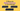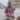# 3 Ways to Detect an Array in JavaScript

Posted June 30, 2020Start discussion

Checking whether a value is an array in JavaScript is necessary when a variable is expected to be an array, but it could be a plain object or even a primitive.

In this post, you’ll find 3 good ways to detect an array instance in JavaScript.

## 1. Array.isArray(value)

If you don’t have time to read the entire post, here’s a good way to detect an array in JavaScript: just use `Array.isArray(value)` utility function.

But if you want to know more, let’s continue.

Here are a few examples of using `Array.isArray()`:

`javascript`const array = [1, 2, 3];const object = { message: 'Hello!' };const string = 'Hello!';const empty = null;Array.isArray(array);  // => trueArray.isArray(object); // => falseArray.isArray(string); // => falseArray.isArray(empty);  // => false``

`Array.isArray()` has a good browser support. It’s the recommended way to check for an array in JavaScript.

`Array.isArray(value)` utility function returns `true` if `value` is an array.

## 2. value instanceof Array

An array is an object. And like any object in JavaScript, the array instance has a constructor function — `Array`.

`Array` invoked as a constructor (prefixed with `new` keyword) creates array instances:

`javascript`const array = new Array(1, 2, 3);array; // => [1, 2, 3]``

Moreover, the array instance exposes its constructor using a property `.constructor`:

`javascript`const array = [1, 2, 3];array.constructor === Array; // => true``

What is the operator that verifies whether a function is the constructor of an instance? `instanceof`!

Now emerges the next way to detect an array: `value instanceof Array` evaluates to `true` if `value` is an array.

Let’s see a few examples:

`javascript`const array = [1, 2, 3];const object = { message: 'Hello!' };const string = 'Hello!';const empty = null;array  instanceof Array; // => trueobject instanceof Array; // => falsestring instanceof Array; // => falseempty  instanceof Array; // => false``

Resuming:

`value instanceof Array` expressions evaluates to `true` if `value` is an array.

Note: `value instanceof Array` evaluates to `false` when `value` is an array created in a different iframe than the `Array` constructor function. If you’re not writing cross-frames JavaScript, use this approach without worries.

## 3. ({}).toString.call(value)

`toString()` method of a plain JavaScript object returns `'[object <type>]'`, where `<type>` is the object type it was called upon. Take a look at the specification which indicates how exactly `Object.prototype.toString()` works.

In the simplest case, when `toString()` method is invoked directly on a plain object, it returns `'[object Object]'`:

`javascript`({}).toString(); // => '[object Object]'``

However, you can use an indirect call of `toString()` on different types of values, and the method returns the corresponding string representation of the type:

`javascript`const array = [1, 2, 3];const object = { message: 'Hello' };const string = 'Hello!';const empty = null;({}).toString.call(array);   // => '[object Array]'({}).toString.call(object);  // => '[object Object]'({}).toString.call(string);  // => '[object String]'({}).toString.call(empty);   // => '[object Null]'``

Now you can spot the idea: `({}).toString.call(value)` equals to `'[object Array]'` if `value` is an array.

`javascript`const array = [1, 2, 3];({}).toString.call(array) === '[object Array]'; // => true``

In conclusion:

`({}).toString.call(value) === '[object Array]'` expression evaluates to `true` if `value` is an array.

## 4. Summary

To detect an array my recommendation is to use `Array.isArray(value)`. The function returns a boolean whether `value` is an array. Simple as is.

Another approach is `value instanceof Array` expression that evaluates to `true` if `value` is an array. This approach uses the idea that `Array` function is the constructor of the arrays.

Finally, not the most aesthetic approach is to use the expression `({}).toString.call(value) === '[object Array]'` that is `true` if `value` is an array.

`Object.prototype.toString()` returns the type representation of the object it was invoked upon, and the type representation of arrays is `'[object Array]'`.

What is your favorite way to detect arrays in JavaScript?

## Quality posts into your inbox

I regularly publish posts containing:

• Important JavaScript concepts explained in simple words
• Overview of new JavaScript features
• How to use TypeScript and typing
• Software design and good coding practices